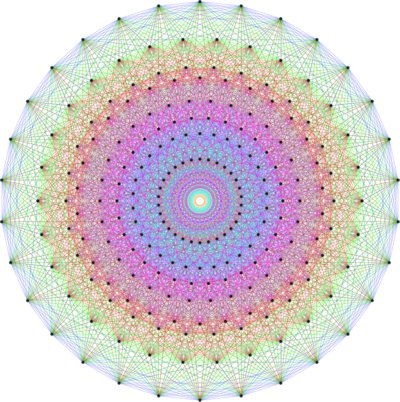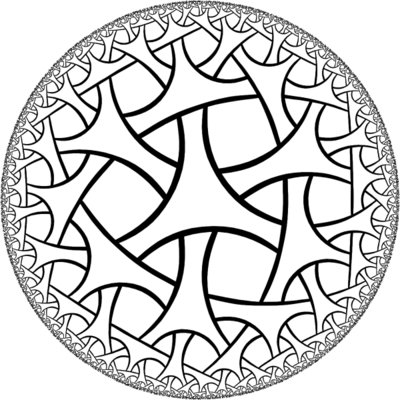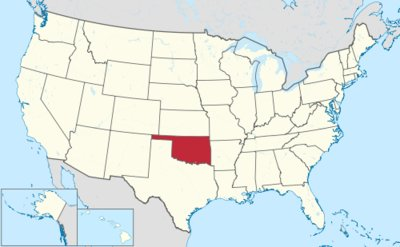# Research in the Math Department

The department is home to world-class research mathematicians in specialties as diverse as mathematics itself. These research areas may be loosely grouped into:

Faculty, graduate students, and undergraduates meet weekly in seminars, detailed on the events page. The main seminars are:

Several members of the department are regular co-organizers of local recurring conference series:

In recognition of its research output, the Carnegie Classification of Institutions of Higher Education designates OU as an R1 university.## Algebra

Algebra is the study of abstract objects that adhere to simple rules, such as number systems, polynomials, vector spaces, and symmetries. These patterns and results have been applied everywhere from Sudoku to string theory. The algebra group meets weekly at the Algebra and Representation Theory Seminar (ARTS) and is involved in the TORA conference series.

### Representation Theory

Representation theory explores mathematical objects by studying the spaces they act upon. It leverages powerful tools from linear algebra, Lie theory, partial differential equations and category theory. It illuminates and generalizes Fourier analysis via harmonic analysis. It is connected to geometry via invariant theory. The subject lies at the heart of the Langlands program, a web of conjectures that guides much of modern number theory. It has many applications in physics and engineering — in particular, to quantum information theory. Members of the representation theory group at OU include:

• Kaan Akin — Representation Theory of Linear Groups and Commutative Algebra.
• Jonathan Kujawa — Lie Theory, Representation Theory, Algebraic Combinatorics.
• András Cristian Lőrincz — Algebraic Geometry, Representation Theory, Commutative Algebra.
• Kimball Martin — Number Theory, Automorphic Forms, Representation Theory.
• Murad Özaydın — Algebraic Topology, Combinatorics.
• Peter Patzt — Group cohomology of arithmetic groups, representation stability, and homological stability.
• Ameya Pitale — Number Theory, Automorphic forms and Representations, Special Values and Integral Representations of L-functions, Siegel Modular Forms.
• Tomasz Przebinda — Representation Theory.
• Alan Roche — Representation Theory of p-adic Groups.

### Number Theory

As old as mathematics itself, number theory studies equations and surprising patterns of integers and prime numbers. Modern number theory integrates geometry, algebra and analysis through objects such as zeta functions, elliptic curves, modular forms and automorphic representations. Members of the number theory group at OU include:

• Kimball Martin — Number Theory, Automorphic Forms, Representation Theory.
• Ameya Pitale — Number Theory, Automorphic forms and Representations, Special Values and Integral Representations of L-functions, Siegel Modular Forms.
• Alan Roche — Representation Theory of p-adic Groups.

### Algebraic Geometry

At its most basic level, algebraic geometry is simply the study of systems of polynomial equations. But more broadly it is the art of using algebraic tools to study geometric objects and using geometric tools to study algebraic objects. As such, it has evolved in close relation to several other areas of mathematics, most notably number theory, representation theory, algebraic combinatorics, and complex geometry. Members of the algebraic geometry group at OU include:

• Roi Docampo — Algebraic Geometry, Singularity Theory.
• Lucy Lifschitz — Structure, representations and actions of arithmetic groups, Geometric and Measured Group Theory.
• András Cristian Lőrincz — Algebraic Geometry, Representation Theory, Commutative Algebra.
• Travis Mandel — I am interested in the interplay of algebraic geometry, mirror symmetry, tropical geometry, Gromov-Witten theory, and cluster algebras.
• Greg Muller — Cluster Algebras, Combinatorics.

### Combinatorics

Combinatorics is a catch-all term that encompasses the study of a wide array of discrete structures such as graphs, networks, and counting problems. Combinatorics is widely used in computer science, from algorithm design to error-correction. Members of the combinatorics group at OU include:

#### Related Faculty to Algebra

• Boris Apanasov — Varieties of Group Representations, Combinatorics of Tessellations and Group Actions, Application of Group Growth in Analysis.
• Michael Jablonski — Riemannian geometry, geometry of homogeneous metrics, Einstein and Ricci soliton metrics.
• Justin Malestein — Mapping Class Groups, Discrete Geometry, Low-Dimensional Topology, Geometric Group Theory.
• Ricardo Mendes — Symmetry of Riemannian manifolds, in particular polar group actions; Non-negative sectional curvature and a variant called strongly non-negative curvature; Singular Riemannian foliations, especially in round spheres; Minimal surfaces.

#### Emeritus Faculty in Algebra## Analysis/Applied Math

Analysis is the study of the infinitesimal behavior of functions, such as limits, integration, and other tools from calculus. The ubiquity of differential equations in scientific models makes tools from analysis some of the most important in applications.

### Analysis

The analysis group meets weekly at the Analysis Seminar, and features working groups specializing in convexity theory, partial differential equations, and the theory of frames. Members of the analysis group at OU include:

• John Albert — Partial Differential Equations.
• Javier Alejandro Chávez-Domínguez — Banach Spaces, Nonlinear Functional Analysis and Operator Spaces.
• Alexander Grigo — Probability, statistical mechanics, dynamical systems.
• Yan Mary He — Complex dynamics, thermodynamic formalism, Kleinian groups and hyperbolic geometry.
• Keri Kornelson — Harmonic and functional analysis, particularly frames, wavelets, and iterated function systems.
• Nikola Petrov — Dynamical systems; Applications of analysis, geometry, and stochastic processes to natural phenomena.
• Tomasz Przebinda — Representation Theory.
• Christian Remling — Spectral Theory, mathematical physics.
• Weinan Wang — Partial differential equations; fluid dynamics; kinetic models; mathematical biology; stochastic partial differential equations.
• Shihshu Walter Wei — Geometric Analysis, Several Complex Variables, Geometric Function Theory, Nonlinear Potential Theory, Geometric Measure Theory, Calculus of Variations.
• Yilun (Allen) Wu — Calculus of variations, bifurcation theory, nonlinear dispersive equations, inverse scattering transforms, with applications in fluid mechanics and stellar structure.
• Pengfei Zhang — Smooth Ergodic Theory.
• Meijun Zhu — Partial Differential Equations, Calculus of Variations.

### Applied Math

The applied math group meets weekly at the Dynamical Systems Working Seminar and the Applied Math Seminar, and features specialties in dynamical systems and partial differential equations. Members of the applied math group at OU include:

• Alexander Grigo — Probability, statistical mechanics, dynamical systems.
• Miroslav Kramar — Topological data analysis: computational algebraic topology, analysis of non-linear structures in high-dimensional data, manifold reconstruction, persistent homology. Non-linear dynamics: reconstruction of dynamics from data, pattern formation, Conley index theory, anomaly detection. Interdisciplinary research: dense granular media, turbulent flows, dynamics of silo deformation, protein structure and function, spreading processes on networks.
• Nikola Petrov — Dynamical systems; Applications of analysis, geometry, and stochastic processes to natural phenomena.
• Weinan Wang — Partial differential equations; fluid dynamics; kinetic models; mathematical biology; stochastic partial differential equations.
• Ying Wang — Scientific Computing, Mathematical Modeling, Applied Analysis, Hyperbolic Conservation Laws, Mathematical Biology, Image Processing, Computational Linear Algebra and Operations Research.

#### Related Faculty to Analysis and Applied Math

• Boris Apanasov — Geometric Analysis, Quasiconformal/​Quasisymmetric/​Quasiregular Maps in Symmetric Spaces, Dynamics/​Ergodicity of discrete group action, Application of Group Growth in Analysis.
• Murad Özaydın — Algebraic Topology, Combinatorics.

#### Analysis and Applied Math Post-Docs

• Jack Arbunich — Nonlinear Partial Differential Equations and Mathematical Physics.
• Victor Bailey — Harmonic Analysis and Operator Theory.
• Gary Moon — Nonlinear Partial Differential Equations, Fluid Dynamics, Free Boundary Problems and Microlocal Analysis.

#### Emeritus Faculty in Analysis and Applied Math## Geometry/Topology

Geometry is the study of distance, length, angles, geodesics etc., but in a modern context this includes the study of these attributes on manifolds (e.g., surfaces, 3-space) with positive or negative curvature and on more combinatorial objects. Topology, in part, is the study of continuous deformations and transformations and properties of spaces which are preserved by such deformations and transformations. The geometry/topology group meets weekly at the Geometry and Topology Seminar, and is involved in the organization of the Redbud Topology Conference and the Midwest Geometry Conference. Our Geometry/Topology group has a few main areas of focus: geometric group theory, low-dimensional/geometric topology, Riemannian geometry and algebraic topology.

### Geometric Group Theory and Low-dimensional/Geometric Topology

Broadly speaking, geometric group theory is the study of groups, often infinite and discrete, from geometric properties of the group itself or of its action on geometric objects. Low-dimensional topology is the study of manifolds of 2, 3, or 4 dimensions. Some particular topics studied by our faculty are growth rate in groups, Dehn filling functions, stable commutator length, mapping class groups, and Teichmüller geometry.

• Boris Apanasov — Geometry/​Topology of Real/​Complex Hyperbolic Manifolds, Teichmuller Spaces of 3&4-Manifolds, Geometric 4-cobordisms/​Homology Cobordisms, Fibre Bundles & Almost Nilpotent Manifolds, Conformal/​Contact/​CR Manifolds.
• Noel Brady — Geometric Group Theory.
• Max Forester — Geometric Group Theory, Low-Dimensional Topology.
• Yan Mary He — Complex dynamics, thermodynamic formalism, Kleinian groups and hyperbolic geometry.
• Justin Malestein — Mapping Class Groups, Discrete Geometry, Low-Dimensional Topology, Geometric Group Theory.
• Nicholas Miller — Hyperbolic Geometry, Low Dimensional Topology, Homogeneous Dynamics, and Arithmetic Groups.
• Peter Patzt — Group cohomology of arithmetic groups, representation stability, and homological stability.
• Jing Tao — Teichmuller Theory, Low-dimensional Topology, Geometric Group Theory.

### Riemannian Geometry

Riemannian geometry is the study of manifolds and the geometry and curvature of their Riemannian metrics. Some particular topics studied by our faculty are isometry groups, hyperbolic geometry, manifolds with nonnegative curvature, nilpotent/solvable Lie groups, and Einstein and Ricci soliton metrics.

• Boris Apanasov — Geometry of Locally Symmetric Spaces of Rank 1, Discrete Group Action, Real/​Complex/​Quaternionic Hyperbolic Geometry, Nilpotent/​Contact/​CR Geometry.
• Michael Jablonski — Riemannian geometry, geometry of homogeneous metrics, Einstein and Ricci soliton metrics.
• Ricardo Mendes — Symmetry of Riemannian manifolds, in particular polar group actions; Non-negative sectional curvature and a variant called strongly non-negative curvature; Singular Riemannian foliations, especially in round spheres; Minimal surfaces.
• Shihshu Walter Wei — Riemannian Geometry, Complex Geometry, Finsler Geometry, p-Harmonic Maps, Minimal Varieties, Gauge Theory, Curvature and Topology, Transformation Groups.

### Algebraic Topology

Algebraic topology is the study of algebraic invariants of topological spaces and the study of algebraic objects from a topological perspective.

• Murad Özaydın — Algebraic Topology, Combinatorics.
• Peter Patzt — Group cohomology of arithmetic groups, representation stability, and homological stability.

### Topological Data Analysis

Topological Data Analysis (TDA) provides a mathematical framework for identifying and describing relevant features of complex and possibly high dimensional data. In many applications data is sampled from an unknown manifold. The methods of TDA allow us to study the shape of the manifold from its finite sample. The shape is described by topological invariants which can be computed using algebraic topology.

• Miroslav Kramar — Topological data analysis: computational algebraic topology, analysis of non-linear structures in high-dimensional data, manifold reconstruction, persistent homology. Non-linear dynamics: reconstruction of dynamics from data, pattern formation, Conley index theory, anomaly detection. Interdisciplinary research: dense granular media, turbulent flows, dynamics of silo deformation, protein structure and function, spreading processes on networks.
• Murad Özaydın — Algebraic Topology, Combinatorics.

#### Related Faculty to Geometry/Topology

• Lucy Lifschitz — Structure, representations and actions of arithmetic groups, Geometric and Measured Group Theory.

#### Emeritus Faculty in Geometry/Topology## Research in Undergraduate Mathematics Education (RUME)

The RUME (Research in Undergraduate Mathematics Education) program at OU offers a PhD in mathematics with research emphasizing mathematics education. The RUME group meets weekly at the RUME seminar, and features research specializations in linear algebra instruction, conceptualization of proofs, and active-learning techniques.

Faculty affiliated with the RUME program include:

• Deborah Moore-Russo — Research in Undergraduate Mathematics Education (especially, understanding of slope and vectors); Teacher Reflection; Digital Tools & Spaces in STEM learning communities; First-Year Mathematics Policies, Procedures, Supports, and Services.
• Milos Savic — Math Education: investigating mathematical creativity in undergraduate classrooms, including calculus and proof-based courses.
• Sepideh Stewart — Research in Mathematics Education at University Level.

#### Related Faculty to RUME

• Keri Kornelson — Harmonic and functional analysis, particularly frames, wavelets, and iterated function systems.

#### Emeritus Faculty in RUME## Conference Series

### Midwest Geometry Conference

The Midwest Geometry Conference is an annual meeting in topics related to differential geometry and geometric analysis. Members of the department have been frequently involved in its organization since its founding in 1991. See here for an overview of its history until 2015. Recent installments: 2016, 2017, 2019.

### Redbud Topology Conference

The Redbud Topology conference series is a recurring regional conference in topology and related areas, hosted in rotation by the University of Arkansas, Oklahoma State University, and the University of Oklahoma.

### TORA

TORA stands for "Texas-Oklahoma Representations and Automorphic forms", and is a conference series hosted in rotation by Oklahoma State University, the University of Oklahoma, and the University of North Texas.